Run this tutorial

Click here to run this tutorial on mybinder.org:Please note that starting the notebook server may take a couple of minutes.

# Tutorial: Model order reduction for PDE-constrained optimization problems¶

A typical application of model order reduction for PDEs are PDE-constrained parameter optimization problems. These problems aim to find a local minimizer of an objective functional depending on an underlying PDE which has to be solved for all evaluations. A prototypical example of a PDE-constrained optimization problem can be defined in the following way. For a physical domain $$\Omega \subset \mathbb{R}^d$$ and a parameter set $$\mathcal{P} \subset \mathbb{R}^P$$, we want to find a solution of the minimization problem

$\min_{\mu \in \mathcal{P}} J(u_{\mu}, \mu), \tag{P.a}$

where $$u_{\mu} \in V := H^1_0(\Omega)$$ is the solution of

$\begin{equation} \label{eq:primal} a_{\mu}(u_{\mu}, v) = f_{\mu}(v) \qquad \forall \, v \in V \tag{P.b}. \end{equation}$

The equation $$\eqref{eq:primal}$$ is called the primal equation and can be arbitrarily complex. MOR methods in the context of PDE-constrained optimization problems thus aim to find a surrogate model of $$\eqref{eq:primal}$$ to reduce the computational costs of an evaluation of $$J(u_{\mu}, \mu)$$.

If there exists a unique solution $$u_{\mu}$$ for all $$\mu \in \mathcal{P}$$, we can rewrite § by using the so-called reduced objective functional $$\mathcal{J}(\mu):= J(u_{\mu}, \mu)$$ leading to the equivalent problem: Find a solution of

$\min_{\mu \in \mathcal{P}} \mathcal{J}(\mu). \tag{\hat{P}}$

There exist plenty of different methods to solve ($$\hat{P}$$) by using MOR methods. Some of them rely on an RB method with traditional offline/online splitting, which typically result in a very online efficient approach. Recent research also tackles overall efficiency by overcoming the expensive offline phase, which we will discuss further below.

In this tutorial, we use a simple linear scalar valued objective functional and an elliptic primal equation to compare different approaches that solve ($$\hat{P}$$).

## An elliptic model problem with a linear objective functional¶

We consider a domain $$\Omega:= [-1, 1]^2$$, a parameter set $$\mathcal{P} := [0,\pi]^2$$ and the elliptic equation

$- \nabla \cdot \big( \lambda(\mu) \nabla u_\mu \big) = l$

with data functions

\begin{split}\begin{align} l(x, y) &= \tfrac{1}{2} \pi^2 \cos(\tfrac{1}{2} \pi x) \cos(\tfrac{1}{2} \pi y),\\ \lambda(\mu) &= \theta_0(\mu) \lambda_0 + \theta_1(\mu) \lambda_1,\\ \theta_0(\mu) &= 1.1 + \sin(\mu_0)\mu_1,\\ \theta_1(\mu) &= 1.1 + \sin(\mu_1),\\ \lambda_0 &= \chi_{\Omega \backslash \omega},\\ \lambda_1 &= \chi_\omega,\\ \omega &:= [-\tfrac{2}{3}, -\tfrac{1}{3}]^2 \cup ([-\tfrac{2}{3}, -\tfrac{1}{3}] \times [\tfrac{1}{3}, \tfrac{2}{3}]). \end{align}\end{split}

The diffusion is thus given as the linear combination of scaled indicator functions where $$\omega$$ is defined by two blocks in the left half of the domain, roughly where the w is here:

+-----------+
|           |
|  w        |
|           |
|  w        |
|           |
+-----------+


From the definition above we can easily deduce the bilinear form $$a_{\mu}$$ and the linear functional $$f_{\mu}$$ for the primal equation. Moreover, we consider the linear objective functional

$\mathcal{J}(\mu) := \theta_{\mathcal{J}}(\mu)\, f_\mu(u_\mu)$

where $$\theta_{\mathcal{J}}(\mu) := 1 + \frac{1}{5}(\mu_0 + \mu_1)$$.

With this data, we can construct a StationaryProblem in pyMOR.

from pymor.basic import *
import numpy as np

domain = RectDomain(([-1,-1], [1,1]))
indicator_domain = ExpressionFunction(
'(-2/3. <= x) * (x <= -1/3.) * (-2/3. <= x) * (x <= -1/3.) * 1. \
+ (-2/3. <= x) * (x <= -1/3.) *  (1/3. <= x) * (x <=  2/3.) * 1.',
dim_domain=2)
rest_of_domain = ConstantFunction(1, 2) - indicator_domain

l = ExpressionFunction('0.5*pi*pi*cos(0.5*pi*x)*cos(0.5*pi*x)', dim_domain=2)

parameters = {'diffusion': 2}
thetas = [ExpressionParameterFunctional('1.1 + sin(diffusion)*diffusion', parameters,
derivative_expressions={'diffusion': ['cos(diffusion)*diffusion',
'sin(diffusion)']}),
ExpressionParameterFunctional('1.1 + sin(diffusion)', parameters,
derivative_expressions={'diffusion': ['0',
'cos(diffusion)']}),

]
diffusion = LincombFunction([rest_of_domain, indicator_domain], thetas)

theta_J = ExpressionParameterFunctional('1 + 1/5 * diffusion + 1/5 * diffusion', parameters,
derivative_expressions={'diffusion': ['1/5','1/5']})

problem = StationaryProblem(domain, l, diffusion, outputs=[('l2', l * theta_J)])


We now use pyMOR’s builtin discretization toolkit (see Tutorial: Using pyMOR’s discretization toolkit) to construct a full order StationaryModel. Since we intend to use a fixed energy norm

$\|\,.\|_{\bar{\mu}} : = a_{\,\bar{\mu}}(.,.),$

we also define $$\bar{\mu}$$, which we pass via the argument mu_energy_product. Also, we define the parameter space $$\mathcal{P}$$ on which we want to optimize.

mu_bar = problem.parameters.parse([np.pi/2,np.pi/2])

fom, data = discretize_stationary_cg(problem, diameter=1/50, mu_energy_product=mu_bar)
parameter_space = fom.parameters.space(0, np.pi)


We now define the first function for the output of the model that will be used by the minimizer.

def fom_objective_functional(mu):
return fom.output(mu)[0, 0]


We also pick a starting parameter for the optimization method, which in our case is $$\mu^0 = (0.25, 0.5)$$.

initial_guess = [0.25, 0.5]


Next, we visualize the diffusion function $$\lambda_\mu$$ by using InterpolationOperator for interpolating it on the grid.

from pymor.discretizers.builtin.cg import InterpolationOperator

diff = InterpolationOperator(data['grid'], problem.diffusion).as_vector(fom.parameters.parse(initial_guess))
fom.visualize(diff)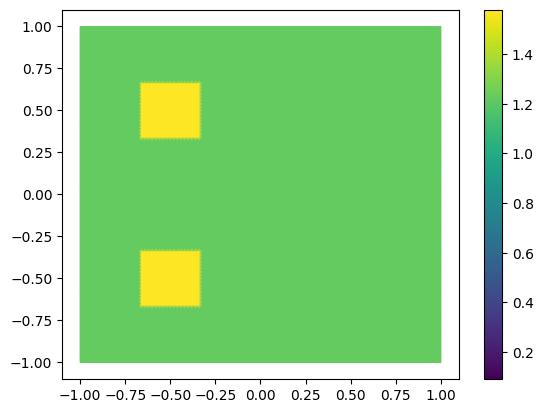print(data['grid'])

Tria-Grid on domain [-1,1] x [-1,1]
x0-intervals: 100, x1-intervals: 100
elements: 40000, edges: 60200, vertices: 20201


We can see that our FOM model has 20201 DoFs which just about suffices to resolve the data structure in the diffusion. This suggests to use an even finer mesh. However, for enabling a faster runtime for this tutorial, we stick with this mesh and remark that refining the mesh does not change the interpretation of the methods that are discussed below. It rather further improves the speedups achieved by model reduction.

Before we discuss the first optimization method, we define helpful functions for visualizations.

import matplotlib as mpl
mpl.rcParams['figure.figsize'] = (12.0, 8.0)
mpl.rcParams['font.size'] = 12
mpl.rcParams['savefig.dpi'] = 300
mpl.rcParams['figure.subplot.bottom'] = .1
mpl.rcParams['axes.facecolor'] = (0.0, 0.0, 0.0, 0.0)

from mpl_toolkits.mplot3d import Axes3D # required for 3d plots
from matplotlib import cm # required for colors

import matplotlib.pyplot as plt
from time import perf_counter

def compute_value_matrix(f, x, y):
f_of_x = np.zeros((len(x), len(y)))
for ii in range(len(x)):
for jj in range(len(y)):
f_of_x[ii][jj] = f((x[ii], y[jj]))
x, y = np.meshgrid(x, y)
return x, y, f_of_x

def plot_3d_surface(f, x, y, alpha=1):
X, Y = x, y
fig = plt.figure()
x, y, f_of_x = compute_value_matrix(f, x, y)
ax.plot_surface(x, y, f_of_x, cmap='Blues',
linewidth=0, antialiased=False, alpha=alpha)
ax.view_init(elev=27.7597402597, azim=-39.6370967742)
ax.set_xlim3d([-0.10457963, 3.2961723])
ax.set_ylim3d([-0.10457963, 3.29617229])
return ax

def addplot_xy_point_as_bar(ax, x, y, color='orange', z_range=None):
ax.plot([y, y], [x, x], z_range if z_range else ax.get_zlim(), color)


Now, we can visualize the objective functional on the parameter space

ranges = parameter_space.ranges['diffusion']
XX = np.linspace(ranges + 0.05, ranges, 10)

plot_3d_surface(fom_objective_functional, XX, XX)

<Axes3D: >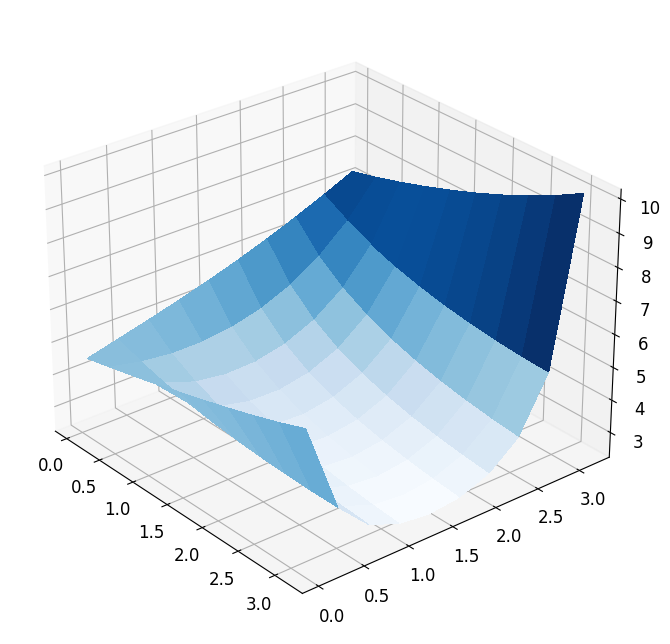Taking a closer look at the functional, we see that it is at least locally convex with a locally unique minimum. In general, however, PDE-constrained optimization problems are not convex. In our case changing the parameter functional $$\theta_{\mathcal{J}}$$ can already result in a very different non-convex output functional.

In order to record some data during the optimization, we also define helpful functions for recording and reporting the results in this tutorial.

def prepare_data(offline_time=False, enrichments=False):
data = {'num_evals': 0, 'evaluations' : [], 'evaluation_points': [], 'time': np.inf}
if offline_time:
data['offline_time'] = offline_time
if enrichments:
data['enrichments'] = 0
return data

def record_results(function, data, adaptive_enrichment=False, opt_dict=None, mu=None):
# this is for the adaptive case! rom is shiped via the opt_dict argument.
assert opt_dict is not None
QoI, data, rom = function(mu, data, opt_dict)
opt_dict['opt_rom'] = rom
else:
QoI = function(mu)
data['num_evals'] += 1
data['evaluation_points'].append(mu)
data['evaluations'].append(QoI)
return QoI

def report(result, data, reference_mu=None):
if (result.status != 0):
print('\n failed!')
else:
print('\n succeeded!')
print(f'  mu_min:    {fom.parameters.parse(result.x)}')
print(f'  J(mu_min): {result.fun}')
if reference_mu is not None:
print(f'  absolute error w.r.t. reference solution: {np.linalg.norm(result.x-reference_mu):.2e}')
print(f'  num iterations:     {result.nit}')
print(f'  num function calls: {data["num_evals"]}')
print(f'  time:               {data["time"]:.5f} seconds')
if 'offline_time' in data:
print(f'  offline time:       {data["offline_time"]:.5f} seconds')
if 'enrichments' in data:
print(f'  model enrichments:  {data["enrichments"]}')
print('')


## Optimizing with the FOM using finite differences¶

There exist plenty optimization methods, and this tutorial is not meant to discuss the design and implementation of optimization methods. We simply use the minimize function from scipy.optimize and use the builtin L-BFGS-B routine which is a quasi-Newton method that can also handle a constrained parameter space. For the whole tutorial, we define the optimization function as follows.

from functools import partial
from scipy.optimize import minimize

tic = perf_counter()
result = minimize(partial(record_results, J, data, adaptive_enrichment, opt_dict),
initial_guess,
bounds=(ranges, ranges),
options={'ftol': 1e-15, 'gtol': 5e-5})
data['time'] = perf_counter()-tic
return result


It is optional to give an expression for the gradient of the objective functional to the minimize function. In case no gradient is given, minimize just approximates the gradient with finite differences. This is not recommended because the gradient is inexact and the computation of finite differences requires even more evaluations of the primal equation. Here, we use this approach for a simple demonstration.

reference_minimization_data = prepare_data()

fom_result = optimize(fom_objective_functional, reference_minimization_data, ranges)

reference_mu = fom_result.x

report(fom_result, reference_minimization_data)

 succeeded!
mu_min:    {diffusion: [1.4246801734272179, 3.141592653589793]}
J(mu_min): 2.3917078763077035
num iterations:     7
num function calls: 27
time:               3.00836 seconds


Taking a look at the result, we see that the optimizer needs $$7$$ iterations to converge, but actually needs $$27$$ evaluations of the full order model. Obviously, this is related to the computation of the finite differences. We can visualize the optimization path by plotting the chosen points during the minimization.

reference_plot = plot_3d_surface(fom_objective_functional, XX, XX, alpha=0.5)

for mu in reference_minimization_data['evaluation_points']: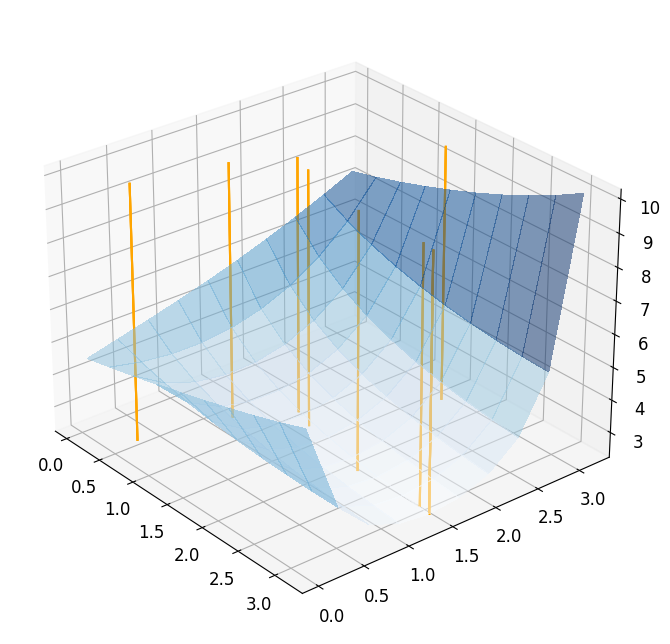## Optimizing with the ROM using finite differences¶

We can use a standard RB method to build a surrogate model for the FOM. As a result, the solution of the primal equation is no longer expensive and the optimization method can evaluate the objective functional quickly. For this, we define a standard CoerciveRBReductor and use the MinThetaParameterFunctional for an estimation of the coerciviy constant.

from pymor.algorithms.greedy import rb_greedy
from pymor.parameters.functionals import MinThetaParameterFunctional
from pymor.reductors.coercive import CoerciveRBReductor

coercivity_estimator = MinThetaParameterFunctional(fom.operator.coefficients, mu_bar)


The online efficiency of MOR methods most likely comes with a rather expensive offline phase. For PDE-constrained optimization, however, it is not meaningful to ignore the offline time of the surrogate model since it can happen that FOM optimization methods would already converge before the surrogate model is even ready. Thus, RB optimization methods (at least for only one configuration) aims for overall efficiency which includes offline and online time. Of course, this effect aggravates if the parameter space is high dimensional because the offline phase can increase even more.

In order to decrease the offline time we guess that we may not require a perfect surrogate model in the sense that a low error tolerance for the rb_greedy already suffices to converge to the same minimum. In our case we choose atol=1e-2 and yield a very low dimensional space. In general, however, it is not a priori clear how to choose atol in order to arrive at a minimum which is close enough to the true optimum.

training_set = parameter_space.sample_uniformly(25)

RB_reductor = CoerciveRBReductor(fom, product=fom.energy_product, coercivity_estimator=coercivity_estimator)
RB_greedy_data = rb_greedy(fom, RB_reductor, training_set, atol=1e-2)

num_RB_greedy_extensions = RB_greedy_data['extensions']
RB_greedy_mus, RB_greedy_errors = RB_greedy_data['max_err_mus'], RB_greedy_data['max_errs']
rom = RB_greedy_data['rom']

print(f'RB system is of size {num_RB_greedy_extensions}x{num_RB_greedy_extensions}')
print(f'maximum estimated model reduction error over training set: {RB_greedy_errors[-1]}')

RB system is of size 3x3
maximum estimated model reduction error over training set: 0.00935354954250117


We can see that greedy algorithm already stops after $$3$$ basis functions. Next, we plot the chosen parameters.

ax = plot_3d_surface(fom_objective_functional, XX, XX, alpha=0.5)

for mu in RB_greedy_mus[:-1]:
mu = mu.to_numpy()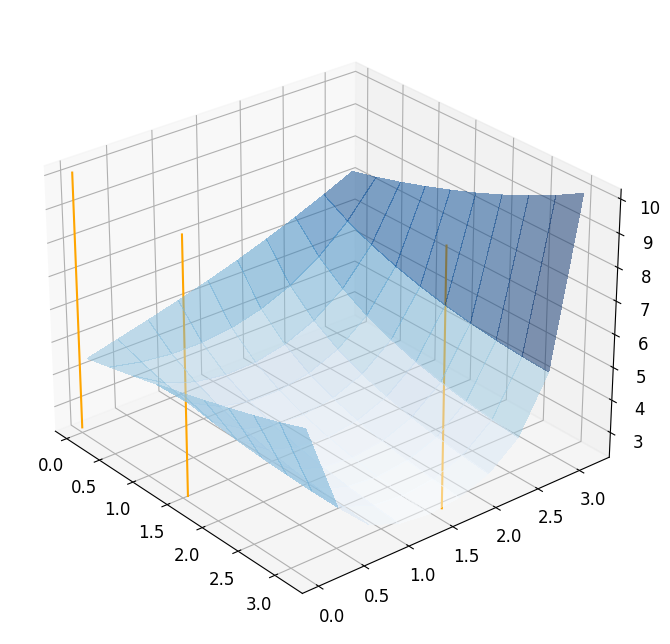Analogously to above, we perform the same optimization method, but use the resulting ROM objective functional.

def rom_objective_functional(mu):
return rom.output(mu)[0, 0]

RB_minimization_data = prepare_data(offline_time=RB_greedy_data['time'])

rom_result = optimize(rom_objective_functional, RB_minimization_data, ranges)

report(rom_result, RB_minimization_data, reference_mu)

 succeeded!
mu_min:    {diffusion: [1.424654211984247, 3.141592653589793]}
J(mu_min): 2.3917078350963017
absolute error w.r.t. reference solution: 2.60e-05
num iterations:     7
num function calls: 27
time:               0.02134 seconds
offline time:       1.97065 seconds


Comparing the result to the FOM model, we see that the number of iterations and evaluations of the model are equal. As expected, we see that the optmization routine is very fast because the surrogate enables almost instant evaluations of the primal equation.

As mentioned above, we should not forget that we required the offline time to build our surrogate. In our case, the offline time is still low enough to get a speed up over the FOM optimization. Luckily, atol=1e-2 was enough to achieve an absolute error of roughly 1e-06 but it is important to notice that we do not know this error before choosing atol.

To show that the ROM optimization roughly followed the same path as the FOM optimization, we visualize both of them in the following plot.

reference_plot = plot_3d_surface(fom_objective_functional, XX, XX, alpha=0.5)
reference_plot_mean_z_lim = 0.5*(reference_plot.get_zlim() + reference_plot.get_zlim())

for mu in reference_minimization_data['evaluation_points']:
z_range=(reference_plot.get_zlim(), reference_plot_mean_z_lim))

for mu in RB_minimization_data['evaluation_points']:
z_range=(reference_plot_mean_z_lim, reference_plot.get_zlim()))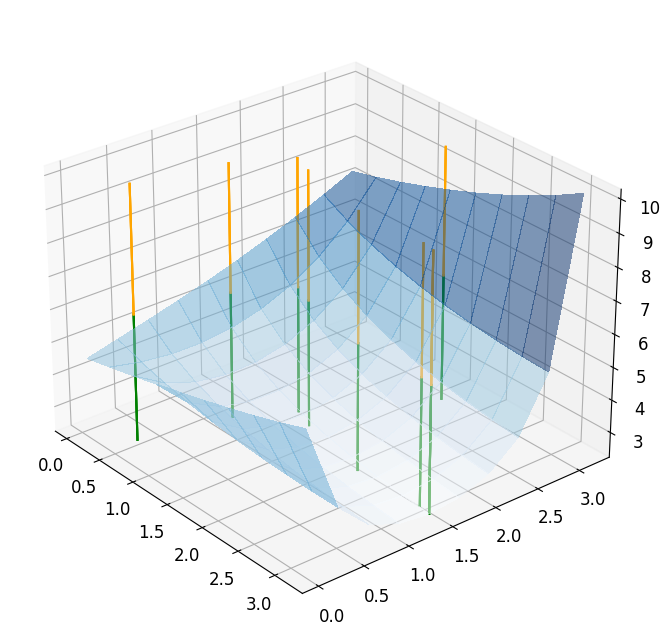## Computing the gradient of the objective functional¶

A major issue of using finite differences for computing the gradient of the objective functional is the number of evaluations of the objective functional. In the FOM example from above we saw that many evaluations of the model were only due to the computation of the finite differences. If the problem is more complex and the mesh is finer, this can lead to a serious waste of computational time. Also from an optimizational point of view it is always better to compute the true gradient of the objective functional.

For computing the gradient of the linear objective functional $$\mathcal{J}(\mu)$$, we can write for every direction $$i= 1, \dots, P$$

\begin{align} \label{gradient:sens} \tag{1} d_{\mu_i} \mathcal{J}(\mu) = \partial_{\mu_i} J(u_{\mu}, \mu) + \partial_u J(u_{\mu}, \mu)[d_{\mu_i} u_{\mu}] = \partial_{\mu_i} J(u_{\mu}, \mu) + J(d_{\mu_i} u_{\mu}, \mu) \end{align}

Thus, we need to compute the derivative of the solution $$u_{\mu}$$ (also called sensitivity). For this, we need to solve another equation: Find $$d_{\mu_i} u_{\mu} \in V$$, such that

$\label{sens} \tag{2} a_\mu(d_{\mu_i} u_{\mu}, v) = \partial_{\mu_i} r_\mu^{\text{pr}}(u_{\mu})[v] \qquad \qquad \forall v \in V$

where $$r_\mu^{\text{pr}}$$ denotes the residual of the primal equation, i.e.

$r_\mu^{\text{pr}}(u)[v] := l_\mu(v) - a_\mu(u, v) \qquad \qquad \text{for all }v \in V$

A major issue of this approach is that the computation of the full gradient requires $$P$$ solutions of $$\eqref{sens}$$. Especially for high dimensional parameter spaces, we can instead use an adjoint approach to reduce the computational cost to only one solution of an additional problem.

The adjoint approach relies on the Lagrangian of the objective functional

$\mathcal{L}(u, \mu, p) = J(u, \mu) + r_\mu^{\text{pr}}(u, p)$

where $$p \in V$$ is the adjoint variable. Deriving optimality conditions for $$\mathcal{L}$$, we end up with the dual equation: Find $$p_{\mu} \in V$$, such that

$\label{dual} \tag{3} a_\mu(v, p_\mu) = \partial_u J(u_\mu, \mu)[v] = J(v, \mu)$

Note that in our case, we then have $$\mathcal{L}(u_{\mu}, \mu, p_{\mu}) = J(u, \mu)$$ because the residual term $$r_\mu^{\text{pr}}(u_{\mu}, p_{\mu})$$ vanishes since $$u_{\mu}$$ solves $$\eqref{eq:primal}$$ and $$p_{\mu}$$ is in the test space $$V$$. By using the solution of the dual problem, we can then derive the gradient of the objective functional by

\begin{split}\begin{align} d_{\mu_i} \mathcal{J}(\mu) &= \partial_{\mu_i} J(u_{\mu}, \mu) + \partial_u J(u_{\mu}, \mu)[d_{\mu_i} u_{\mu}] \\ &= \partial_{\mu_i} J(u_{\mu}, \mu) + a_\mu(d_{\mu_i} u_{\mu}, p_\mu) \\ &= \partial_{\mu_i} J(u_{\mu}, \mu) + \partial_{\mu_i} r_\mu^{\text{pr}}(d_{\mu_i} u_{\mu})[p_\mu] \end{align}\end{split}

We conclude that we only need to solve for $$u_{\mu}$$ and $$p_{\mu}$$ if we want to compute the gradient with the adjoint approach. For more information on this approach we refer to Section 1.6.2 in [HPUU08].

We now intend to use the gradient to speed up the optimization methods from above. All technical requirements are already available in pyMOR.

## Optimizing using a gradient in FOM¶

We can easily include a function to compute the gradient to minimize. Since we use a linear operator and a linear objective functional, the use_adjoint argument is automatically enabled. Note that using the (more general) implementation use_adjoint=False results in the exact same gradient but lacks computational speed. Moreover, the function output_d_mu returns a dict w.r.t. the parameters as default. In order to use the output for minimize we thus use the return_array=True argument.

def fom_gradient_of_functional(mu):

opt_fom_minimization_data = prepare_data()

opt_fom_result = optimize(fom_objective_functional, opt_fom_minimization_data, ranges,

# update the reference_mu because this is more accurate!
reference_mu = opt_fom_result.x

report(opt_fom_result, opt_fom_minimization_data)

 succeeded!
mu_min:    {diffusion: [1.4246556963614603, 3.141592653589793]}
J(mu_min): 2.3917078762139408
num iterations:     7
num function calls: 9
time:               3.01924 seconds


With respect to the FOM result with finite differences, we see that we have a saved the evaluations for computing the gradient. Of course it is also not for free to compute the gradient, but since we are using the dual approach, this will only scale with the factor 2. Furthermore, we can expect that the result above is more accurate which is why we choose it as the reference parameter.

## Optimizing using a gradient in ROM¶

Obviously, we can also include the gradient of the ROM version of the output functional.

def rom_gradient_of_functional(mu):

opt_rom_minimization_data = prepare_data(offline_time=RB_greedy_data['time'])

opt_rom_result = optimize(rom_objective_functional, opt_rom_minimization_data, ranges,

report(opt_rom_result, opt_rom_minimization_data, reference_mu)

 succeeded!
mu_min:    {diffusion: [1.4246542368580375, 3.141592653589793]}
J(mu_min): 2.391707835095847
absolute error w.r.t. reference solution: 1.46e-06
num iterations:     7
num function calls: 9
time:               0.02545 seconds
offline time:       1.97065 seconds


The online phase is even faster but the offline time of course remains the same. We also conclude that the ROM model eventually gives less speedup by using a better optimization method for the FOM and ROM.

## Beyond the traditional offline/online splitting: enrich along the path of optimization¶

We already figured out that the main drawback for using RB methods in the context of optimization is the expensive offline time to build the surrogate model. In the example above, we overcame this issue by choosing a large tolerance atol. As a result, we cannot be sure that our surrogate model is accurate enough for our purpuses. In other words, either we invest too much time to build an accurate model or we face the danger of reducing with a bad surrogate for the whole parameter space. Thinking about this issue again, it is important to notice that we are solving an optimization problem which will eventually converge to a certain parameter. Thus, it only matters that the surrogate is good in this particular region as long as we are able to arrive at it. This gives hope that there must exist a more efficient way of using RB methods without trying to approximate the FOM across the whole parameter space.

One possible way for advanced RB methods is a reduction along the path of optimization. The idea is that we start with an empty basis and only enrich the model with the parameters that we will arive at. This approach goes beyond the classical offline/online splitting of RB methods since it entirely skips the offline phase. In the following code, we will test this method.

pdeopt_reductor = CoerciveRBReductor(
fom, product=fom.energy_product, coercivity_estimator=coercivity_estimator)


In the next function, we implement the above mentioned way of enriching the basis along the path of optimization.

def enrich_and_compute_objective_function(mu, data, opt_dict):
U = fom.solve(mu)
try:
pdeopt_reductor.extend_basis(U)
data['enrichments'] += 1
except:
print('Extension failed')
opt_rom = pdeopt_reductor.reduce()
QoI = opt_rom.output(mu)
return QoI, data, opt_rom

opt_rom = opt_dict['opt_rom']


With this definitions, we can start the optimization method.

opt_along_path_minimization_data = prepare_data(enrichments=True)

opt_dict = {}
opt_along_path_result = optimize(enrich_and_compute_objective_function,
opt_along_path_minimization_data, ranges,

report(opt_along_path_result, opt_along_path_minimization_data, reference_mu)

 succeeded!
mu_min:    {diffusion: [1.4246556963614518, 3.141592653589793]}
J(mu_min): 2.391707876213646
absolute error w.r.t. reference solution: 8.44e-15
num iterations:     7
num function calls: 9
time:               1.74565 seconds
model enrichments:  9


The computational time looks at least better than the FOM optimization and we are very close to the reference parameter. But we are following the exact same path than the FOM and thus we need to solve the FOM model as often as before (due to the enrichments). The only computational time that we safe is the one for the gradients since we compute the dual solutions with the ROM.

## Adaptively enriching along the path¶

In order to further speedup the above algorithm, we enhance it by only adaptive enrichments of the model. For instance it may happen that the model is already good at the next iteration, which we can easily check by evaluating the standard error estimator which is also used in the greedy algorithm. In the next example we will implement this adaptive way of enriching and set a tolerance which is equal to the one that we had as error tolerance in the greedy algorithm.

pdeopt_reductor = CoerciveRBReductor(
fom, product=fom.energy_product, coercivity_estimator=coercivity_estimator)
opt_rom = pdeopt_reductor.reduce()

def enrich_adaptively_and_compute_objective_function(mu, data, opt_dict):
opt_rom = opt_dict['opt_rom']
primal_estimate = opt_rom.estimate_error(opt_rom.parameters.parse(mu))
if primal_estimate > 1e-2:
print('Enriching the space because primal estimate is {} ...'.format(primal_estimate))
U = fom.solve(mu)
try:
pdeopt_reductor.extend_basis(U)
data['enrichments'] += 1
opt_rom = pdeopt_reductor.reduce()
except:
print('... Extension failed')
else:
print('Do NOT enrich the space because primal estimate is {} ...'.format(primal_estimate))
opt_rom = pdeopt_reductor.reduce()
QoI = opt_rom.output(mu)[0, 0]
return QoI, data, opt_rom

opt_along_path_adaptively_minimization_data = prepare_data(enrichments=True)

opt_dict = {'opt_rom': opt_rom}

Enriching the space because primal estimate is [2.98580267] ...

Enriching the space because primal estimate is [0.08625045] ...

Do NOT enrich the space because primal estimate is [0.00063044] ...
Do NOT enrich the space because primal estimate is [0.00122583] ...
Do NOT enrich the space because primal estimate is [0.00234427] ...
Enriching the space because primal estimate is [0.0135848] ...

Enriching the space because primal estimate is [0.01046669] ...

Do NOT enrich the space because primal estimate is [0.00078205] ...
Do NOT enrich the space because primal estimate is [0.00078226] ...

report(opt_along_path_adaptively_result, opt_along_path_adaptively_minimization_data, reference_mu)

 succeeded!
mu_min:    {diffusion: [1.4246559756652408, 3.141592653589793]}
J(mu_min): 2.3917075561126704
absolute error w.r.t. reference solution: 2.79e-07
num iterations:     7
num function calls: 9
time:               0.67203 seconds
model enrichments:  4


Now, we actually only needed $$4$$ enrichments and ended up with an approximation error of about 1e-07 while getting the highest speed up amongst all methods that we have seen above. Note, however, that this is still dependent on the tolerance atol=1e-2 that we chose without knowing that this tolerance suffices to reach the actual minimum. An easy way around this would be to do one optimization step with the FOM after converging. If this changes anything, the ROM tolerance atol was too large. To conclude, we once again compare all methods that we have discussed in this notebook.

print('FOM with finite differences')
report(fom_result, reference_minimization_data, reference_mu)

print('\nROM with finite differences')
report(rom_result, RB_minimization_data, reference_mu)

report(opt_fom_result, opt_fom_minimization_data, reference_mu)

report(opt_rom_result, opt_rom_minimization_data, reference_mu)

print('\nAlways enrich along the path')
report(opt_along_path_result, opt_along_path_minimization_data, reference_mu)


FOM with finite differences

succeeded!
mu_min:    {diffusion: [1.4246801734272179, 3.141592653589793]}
J(mu_min): 2.3917078763077035
absolute error w.r.t. reference solution: 2.45e-05
num iterations:     7
num function calls: 27
time:               3.00836 seconds

ROM with finite differences

succeeded!
mu_min:    {diffusion: [1.424654211984247, 3.141592653589793]}
J(mu_min): 2.3917078350963017
absolute error w.r.t. reference solution: 1.48e-06
num iterations:     7
num function calls: 27
time:               0.02134 seconds
offline time:       1.97065 seconds

succeeded!
mu_min:    {diffusion: [1.4246556963614603, 3.141592653589793]}
J(mu_min): 2.3917078762139408
absolute error w.r.t. reference solution: 0.00e+00
num iterations:     7
num function calls: 9
time:               3.01924 seconds

succeeded!
mu_min:    {diffusion: [1.4246542368580375, 3.141592653589793]}
J(mu_min): 2.391707835095847
absolute error w.r.t. reference solution: 1.46e-06
num iterations:     7
num function calls: 9
time:               0.02545 seconds
offline time:       1.97065 seconds

Always enrich along the path

succeeded!
mu_min:    {diffusion: [1.4246556963614518, 3.141592653589793]}
J(mu_min): 2.391707876213646
absolute error w.r.t. reference solution: 8.44e-15
num iterations:     7
num function calls: 9
time:               1.74565 seconds
model enrichments:  9

succeeded!
mu_min:    {diffusion: [1.4246559756652408, 3.141592653589793]}
J(mu_min): 2.3917075561126704
absolute error w.r.t. reference solution: 2.79e-07
num iterations:     7
num function calls: 9
time:               0.67203 seconds
model enrichments:  4

assert fom_result.nit == 7
assert opt_along_path_result.nit == 7
assert opt_along_path_minimization_data['num_evals'] == 9
assert opt_along_path_minimization_data['enrichments'] == 9

Download the code: tutorial_optimization.md tutorial_optimization.ipynb